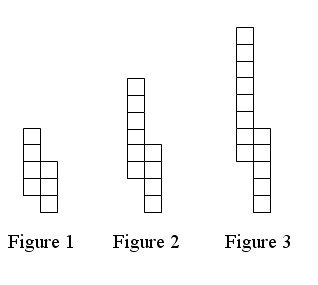### Home > AC > Chapter 3 > Lesson 3.1.5 > Problem3-47

3-47.

On graph paper, draw Figure 0 and Figure 4 for the pattern below. 3-47 HW eTool (CPM) and 3-47 HW eTool (Desmos).1. Represent the number of tiles in each figure in an $x→y$ table. Let $x$ be the figure number and $y$ be the total number of tiles.

1. Use the table to graph the pattern.

How do the figures change as the pattern continues?
Look at your table from part (a).

1. Without drawing Figure 5, predict where its point would lie on the graph. Justify your prediction.

Is there a trend in the way your points lie on the graph?

$(5,19)$; justification may vary.

Use the eTool below to model Figure 0 and Figure 4.
Click the link at right for the full version of the eTool: AC 3-47 HW eTool (CPM Tiles)

Use the eTool below to complete parts (a) and (b).
Click the link at right for the full version of the eTool: AC 3-47 HW eTool (Desmos)